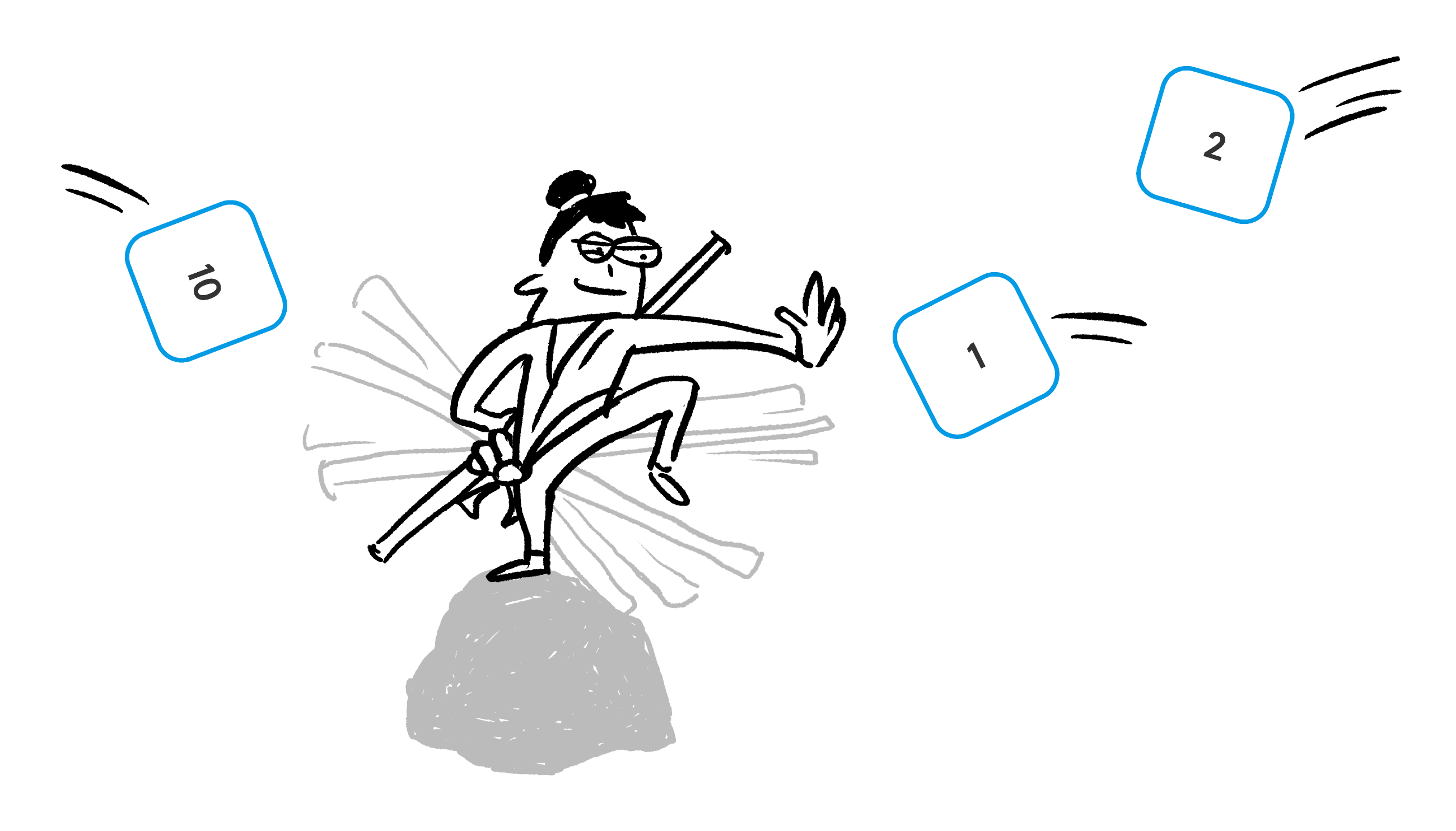### Mathematical Fundamentals

To solve each of these problems, you'll need to drag the number tiles so that every row and column adds up to the target sum beside it. Solving these puzzles takes a combination of algebra and strategic thinking – we'll use puzzles like these to develop problem-solving skills that you can apply to many areas of mathematics.

Give this one a try:

Now try this one.

Solving puzzles that require you to think is a much better way to learn mathematics than drilling rote procedures. You might speed up your arithmetic by drilling, but really understanding and strengthening your skills in algebra is about learning to see what numerical relationships and patterns are hidden within each problem.

In this challenge, you get three copies of each number tile: three $1$-tiles, three $2$-tiles, and three $3$-tiles.

If you’ve ever worked out a Sudoku puzzle, the reasoning that solves the problem should seem very familiar.

The light-grey patches don't get filled in.

Now the target sums have changed...

It is possible to solve this problem.

In each sequence in this course, the challenge-level of the problems increases significantly as you go. This one puzzle skips ahead a few levels, but the solution is animated just below.

Bonus challenge: make the diagonals sum to $15$ as well.

An animation of how you can make one of many possible solutions:

For the bonus puzzle on the previous problem (where every row, column, and diagonal is 15), the main strategy is understanding when and why solutions have specific types of numerical symmetry. In this case, the 5-tile is the "pivot" of the relevant symmetries.

Later in this course, we'll deeply explore the beautiful entanglement of algebra, number theory, and group theory at work in the mathematics of number grids.

This is the last challenge in this sequence, and it's a tough one!

Strategy Tip: If you add up the values on all of the tiles, you'd get that $1+2+3+4+5+6+7+8=36,$ and $36$ is also the product of $12\times 3 = 12+12+12.$

If it's possible, what four numbers wind up in the corners?

We hope that this course's introduction helped you understand and enjoy new mathematical strategies. If at least one problem really made you think, you should consider this experience a success!